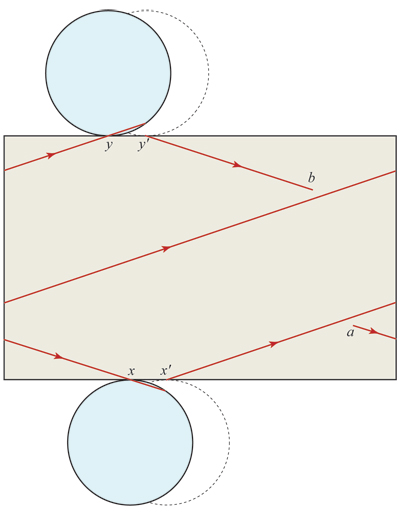# Do elongated convex objects all have long simple geodesics?

Let $S$ be a closed convex surface, the boundary of a compact convex body in $\mathbb{R}^3$. I am interested in whether there are conditions on its shape that ensure that it supports a long, simple (non-self-crossing) geodesic. The length of a geodesic for my purposes is the longest distance you can travel along the geodesic before returning to your starting point. Some condition is necessary for the type of result I seek, for all the geodesics on a sphere have the same length.

Define the elongation $L$ of $S$ as the largest height to diameter ratio, $h/d$, of a cylinder of height $h$ and diameter $d$ in which $S$ is tightly inscribed. By tightly inscribed I mean that $S$ touches the top, bottom, and sides of the cylinder in such a manner that neither the height nor diameter can be reduced.

I could use a theorem of this type:

If $S$ has elongation $L \ge k$, then there is a simple geodesic on $S$ of length $\ge f(k)$, where $f(k)$ is some increasing function of $k$, e.g., $c k$ for a constant $c > 0$.

Perhaps such a theorem cannot exist. Or maybe a theorem of this ilk exists, but only with certain smoothness assumptions? There are always at least three simple closed geodesics on $S$, by a theorem of Lyusternik and Schnirelmann, but perhaps they might all be short?

For an ellipsoid, the three simple closed geodesics follow the major and minor axes, and the longest of those satisfies the type of relationship I seek. (Elongation could as well be defined in terms of an enclosing ellipsoid rather than cylinder.) And a cylindrical $S$ supports a long spiral geodesic:Such spirals are exactly the type of geodesic I seek. Thanks for any ideas or pointers!

Edit. This may not add much, but here is how I view a long geodesic on a cylinder: starting at $a$, crossing the bottom in a segment $x x'$, crossing the top in $y y'$, and stopping at $b$ just before it is about to cross itself.There is a highly relevant paper of Gene Calabi and J. Cao, where they show that there always exists a geodesic of length at least twice the diameter (in the metric space sense) of your surface. I think this answers your question.

• "Simple closed geodesics on convex surfaces," J. Diff. Geom., 1992. Thanks, Igor, this was exactly what I sought! – Joseph O'Rourke Dec 26 '11 at 13:37
• By the way, they attribute the result to Chris Croke. – Igor Rivin Dec 26 '11 at 13:55

Your estimates are not scale invariant, so I am trying to guess what you want from the picture.

A closed geodesic cuts your surface into two discs. Both have geodesic as a boundary, positive curvature and area $\le$ than area of your original surface. If geodesic is long, then (with the intrinsic metric) these discs look almost like segments. It has to have curvature near $\pi$ in concentrated form near the ends.

Thus if long geodesic exist then almost all curvature can be covered by 4 fingers on your surface...

For example,

• you can not have it if Gauss curvature $\ge 1$. (In this case you can still have long shapes: say a doubling of a slice of unit shpere between meridians can be embedded into $\mathbb R^3$ as a convex surface, one can smooth singularities on the poles.)
• you can not have it on a polyhedral surface with more than 4 vertexes. If you have an arbitrary long simple geodesics on the surface of tetrahedral, the sum of angles around each vertex has to be $=\pi$.
• @Anton: Thanks for your remarks, which I am still trying to absorb. On a polyhedral surface, I would instead seek long quasigeodesics, in the sense of Alexandrov. – Joseph O'Rourke Jan 11 '11 at 14:36
• @Anton: Can you please describe for me an elongated shape that has curvature $\ge 1$ at every point? – Joseph O'Rourke Jan 11 '11 at 16:06
• @Joseph: It is in the answer now; BTW the same argument works for quasigeodesics. – Anton Petrunin Jan 11 '11 at 16:44
• @Anton, @Joseph: You guys are talking about "long" geodesics in two different senses. Anton has given conditions under which there is an upper bound for the length of a simple closed geodesic on a particular surface. The tetrahedron has arbitrarily long simple geodesics but not many other surfaces do. Joseph is asking whether for every real number $C > 0$ there is a number $L > 0$ such that for any convex body in $\mathbb E^3$ with diameter$/\sqrt(area) > L$ there is a simple closed geodesic of length$/\sqrt(area) > C$ --- presumably, $C$ should be larger than some constant times $L$. – Bill Thurston Jan 12 '11 at 1:32
• @Bill: Thanks so much for clarifying! I was nonplussed by Anton's obviously knowledgeable comments, and now I know why. But to clarify one point: I am not looking for closed geodesics specifically. Actually a long, simple, geodesic segment is really what I am after. As in my addendum figure. Thanks again. – Joseph O'Rourke Jan 12 '11 at 1:39